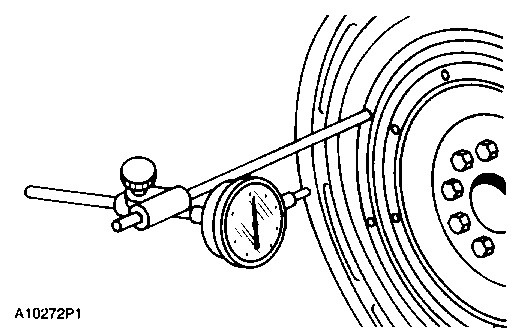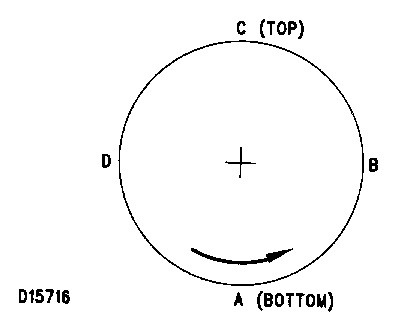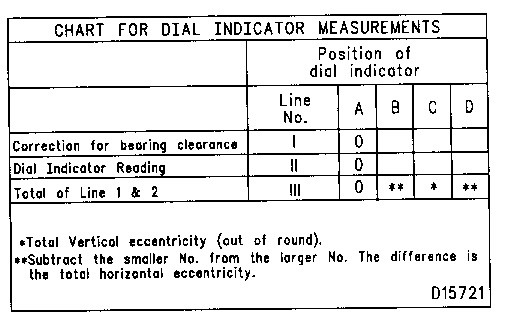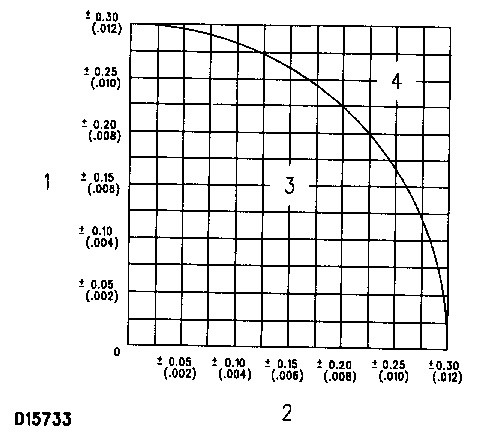# 3116 and 3126 Truck Engines Bore Runout (Radial Eccentricity) Of The Flywheel Housing

1. Fasten the dial indicator as shown so the anvil of the indicator will touch the bore of the flywheel housing.

2. With the dial indicator in position at (C), adjust the dial indicator to “0” (zero). Push the crankshaft up against the top of the bearing. Write the measurement for bearing clearance on line 1 in column (C) in the Chart For Dial Indicator Measurements.8T5096 Dial Indicator Group Installed
(Typical Example)

NOTE: Write the dial indicator measurements with their positive (+) and negative (-) notation (signs). This notation is necessary for making the calculations in the chart correctly.

3. Divide the measurement from Step 2 by 2. Write this number on line 1 in columns (B) & (D).

4. Turn the flywheel to put the dial indicator at (A). Adjust the dial indicator to “0” (zero).

5. Turn the flywheel counterclockwise to put the dial indicator at (B). Write the measurements in the chart.Checking Bore Runout Of The Flywheel Housing

6. Turn the flywheel counterclockwise to put the dial indicator at (C). Write the measurement in the chart.

7. Turn the flywheel counterclockwise to put the dial indicator at (D). Write the measurement in the chart.8. Add lines I & II by columns.

9. Subtract the smaller number from the larger number in line III in columns (B) & (D). The result is the horizontal eccentricity (out of round). Line III, column (C) is the vertical eccentricity.

10. On the graph for total eccentricity, find the point of intersection of the lines for vertical eccentricity and horizontal eccentricity.

11. If the point of intersection is in the range marked “Acceptable”, the bore is in alignment. If the point of intersection is in the range marked “Not Acceptable”, the flywheel housing must be changed.Graph For Total Eccentricity
(1) Total Vertical Eccentricity [mm (in)]. (2) Total Horizontal Eccentricity [mm (in)]. (3) Acceptable. (4) Not Acceptable.